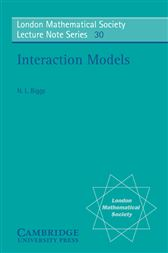Interaction Models

Series: London Mathematical Society Lecture Note Series (No. 30)US\$35.00
US\$31.50
This book is based on a set of lectures given to a mixed audience of physicists and mathematicians. The desire to be intelligible to both groups is the underlying preoccupation of the author. Physicists nowadays are particularly interested in phase transitions. The typical situation is that a system of interacting particles exhibits an abrupt change of behaviour at a certain temperature, although the local forces between the particles are thought to be smooth functions of temperature. This account discusses the theory behind a simple model of such phenomena. An important tool is the mathematical discipline known as the Theory of Graphs. There are five chapters, each subdivided into sections. The first chapter is intended as a broad introduction to the subject, and it is written in a more informal manner than the rest. Notes and references for each chapter are given at the end of the chapter.